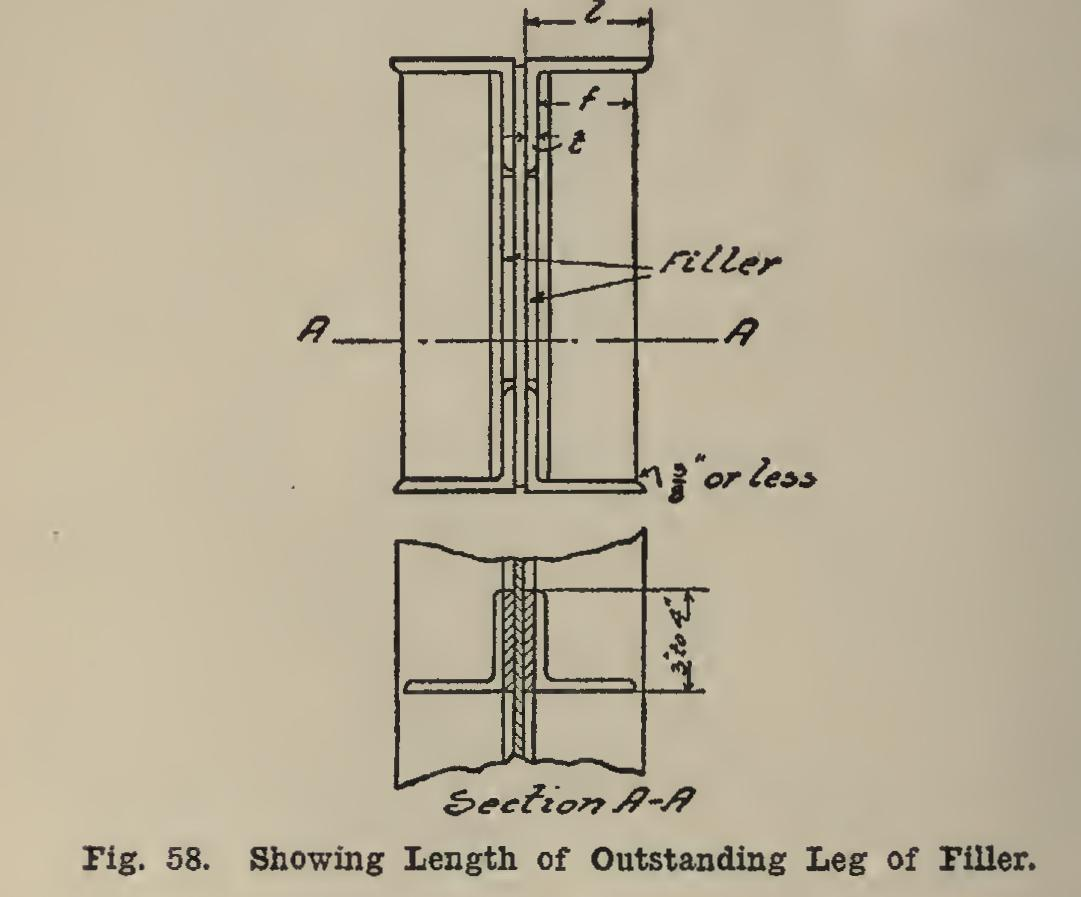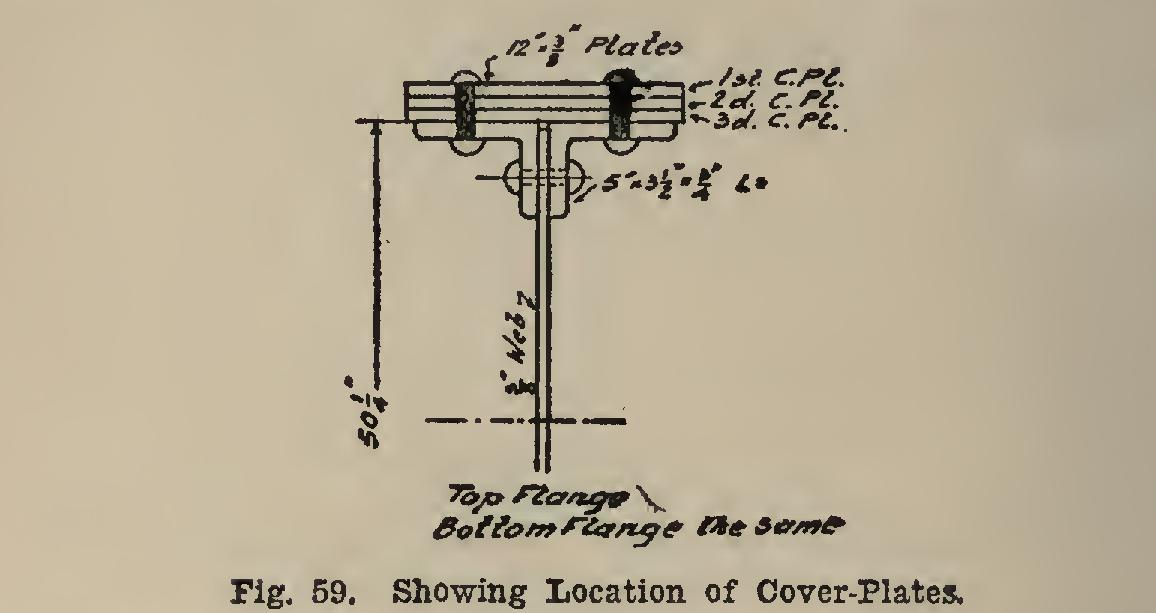# Girders 40

## inch, inches, angles, pounds, cover-plates, cover-plate and leg

Page: 1 2 3 4 5 6 7

Fig. 57 gives a section of the girder as de signed with a cover-plate. The weight of the flanges and web per linear foot are: The design, without cover-plate, weighed 130.2 pounds per linear foot, thus showing a small saving in weight. The extra work necessary to rivet on the cover-plate makes cover-plate sec tions more costly; but the design is better on account of the better riveting together of the outstanding legs of the angles.

The thickness of the end stiffeners of a plate girder should be: For reactions up to 225,000 pounds 2- Inch For reactions from 225,000 pounds -& Inch For reactions from 250,000 to 275,000 pounds Inch For reactions from 275,000 to 300,000 pounds ifr Inch For reactions from 300,000 to 400,000 pounds Inch For reactions over 400,000 pounds Inch The intermediate stiffeners should always be at least inch in thickness. The size of stiffeners should be such that they will extend out as far as possible, and thus support the horizontal leg of the flange angles; and the leg on the web should be such as to get one row of rivets in it. This leg last-mentioned should be from 3 inches to 4 inches. By consulting (C 13-14), it will be noticed that the angles are curved off at the ends, and that this curvature is never greater than inch in any case. From inspection of Fig. 58, it is seen that the outstanding leg of a stiffener cannot be more than: f=1— (t g inch), in which 1 is the outstanding leg of the flange angle; t is the thickness of the flange angle; and inch is the curvature as above explained.For example, suppose that the flange angles were 6 in. by 4 in. by in., the 6-inch leg being horizontal. Here 1=6, and then the stiffener leg cannot be greater than: f=6—(4-14)=5i- inches.

Therefore we can make it 5 inches, and the stiffener angle will be 5 by 3 inches or 5 by 4 inches in size, the thickness depending upon whether it is an end or an intermediate stiffener.

A girder requiring several cover-plates will now be designed in order to show how the cover plates vary. Let the span be 30 feet, the depth of the web-plate 50 inches, and the loads so fixed that the maximum moment is 10,245,900 pound-inches. The allowable unit-stress will be taken as 10,000 pounds per square inch. In order to determine what sized angles will be used, an approximate b will be computed as is done on page 211. It is:

By looking at the 5 by angles and cover-plates of Table XT, it will be seen that if three cover-plates are used, and two 5 by by angles, the area will be 13.87+2X3.75=21.37 square inches. The 5 by angles will be tried.

b.(10,245,900÷10,000)÷(50-1.21) =21.00 square inches required.

If 5 by by 4-inch angles are used with one cover-plate, the area would be 13.87 square inches. Therefore thicker cover-plates are re quired. The amount to be made up by the greater thickness of the cover-plates is 21.00 13.87=7.13 square inches. As inch gives an increase of 0.625 square inch, there will be required 7.13÷0.625=11.4 sixteenths—or as a fraction of a sixteenth cannot be used. This will make inch, or inch, to be added. to the thickness of the cover-plates.

It will be assumed that the weight of :the girder is less than of the load it carries, and that the specifications will in this case allow the weight to be neglected; otherwise the weight must be computed in the same manner as is done on page 211, and the section re-designed. This would only increase the thickness of the cover-plates.The section is as shown in Fig. 59, the web being inch thick. It may be said that unless the depth of the girder is less than of the span, a web will be sufficient. The total net area of the flange is: 3 Cover-plates, 12 by in., at 3.75 each=11.25 sq. in. 2 Angles 5 by 3i by in., at 5.06 each=10.12 sq. in.

Total 21.37 sq. in.° The area of the first cover-plate is 3.75 square inches, and the coefficient is 3.75-÷ 21.37=0.175, say 0.18. This lies about half-way between the ratio values in columns 2 and 3. Therefore the coefficient value will lie between the, coefficient values in columns 2 and 3. The difference between the ratios is 0.20-0.15=0.05, and the difference between the coefficient is 0.45 -0.39=0.06. Now, the difference between the ratio 0.15 and the required ratio is 0.18-0.15= 0.03. Therefore the required coefficient is: 0.03 =0.39+0.036 (say 0.39+0.04) =0.43; and the length of the cover-plate is this value, times the length of the span; or: Length of first 1-in. eover-plate=0.43x30=12.90 feet.

Page: 1 2 3 4 5 6 7